# Pie and Doughnut in WPF Charts (SfChart)

5 Dec 202219 minutes to read

## Pie Chart

`PieSeries` is divided into sectors, illustrating numerical proportion. The following code example illustrates the PieSeries.

``````<chart:PieSeries  XBindingPath="Category"

ItemsSource="{Binding Tax}"

YBindingPath="Percentage"/>``````
``````PieSeries series = new PieSeries()
{

ItemsSource = new ViewModel().Tax,

XBindingPath = "Category",

YBindingPath = "Percentage"

};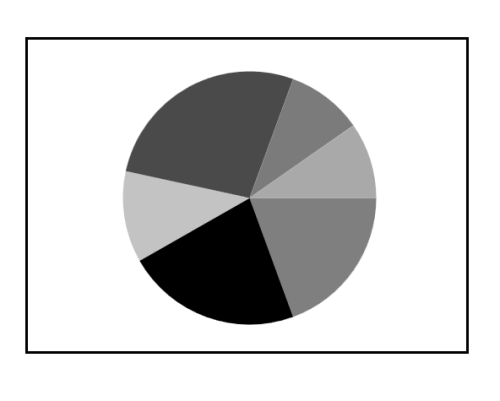The rendering size of the PieSeries can be controlled using `PieCoefficient` property as in below code example.

``````<chart:PieSeries PieCoefficient="0.9"

XBindingPath="Category"

ItemsSource="{Binding Tax}"

YBindingPath="Percentage"/>``````
``````PieSeries series = new PieSeries()
{

ItemsSource = new ViewModel().Tax,

XBindingPath = "Category",

YBindingPath = "Percentage",

PieCoefficient = 0.9

};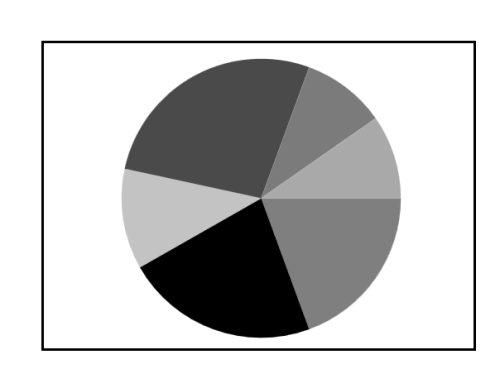### Group small data points into “others”

The small segments in the pie chart can be grouped into the “others” category using the `GroupTo` and `GroupMode` properties of PieSeries.

The `GroupMode` property is used to specify the grouping type based on slice `Angle`, actual data point `Value`, or `Percentage`, and the `GroupTo` property is used to set the limit to group data points into a single slice. The grouped segment is labeled as “Others” in legend and toggled as other segment. The default value of the `GroupTo` property is [`double.NAN`], and the default value of the `GroupMode` property is Value.

Pie series without grouping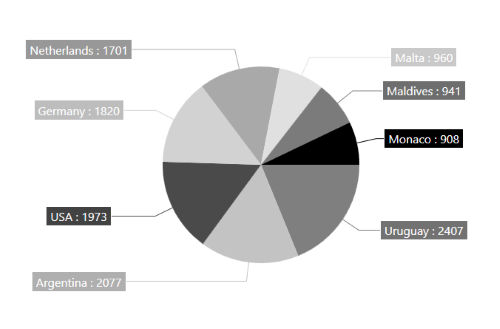Pie series with grouping (Mode - Value)

``````<chart:PieSeries ItemsSource="{Binding Data}" XBindingPath="Country" YBindingPath="Count"
GroupMode="Value" GroupTo="1000" >

ConnectorHeight="80"
ShowLabel="True"
LabelTemplate="{StaticResource DataLabelTemplate}"
SegmentLabelContent="LabelContentPath">
</chart:PieSeries>``````
``````pieSeries.GroupMode = PieGroupMode.Value;
pieSeries.GroupTo = 1000;

{
ShowConnectorLine = true,
ShowLabel = true,
ConnectorHeight = 80,
LabelTemplate = this.RootGrid.Resources["DataLabelTemplate"] as DataTemplate,
SegmentLabelContent = LabelContent.LabelContentPath,
};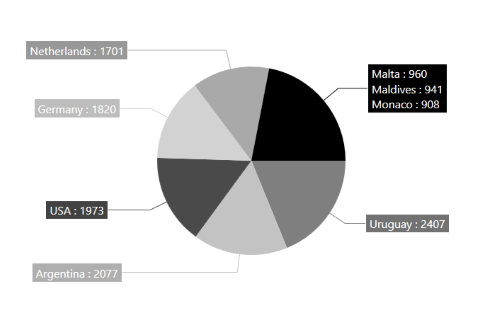Pie series with grouping (Mode - Angle)

``````<chart:PieSeries ItemsSource="{Binding Data}" XBindingPath="Country" YBindingPath="Count"
GroupMode="Angle" GroupTo="30">

ConnectorHeight="80"
ShowLabel="True"
LabelTemplate="{StaticResource DataLabelTemplate}"
SegmentLabelContent="LabelContentPath">
</chart:PieSeries>``````
``````pieSeries.GroupMode = PieGroupMode.Angle;
pieSeries.GroupTo = 30;

{
ShowConnectorLine = true,
ShowLabel = true,
ConnectorHeight = 80,
LabelTemplate = this.RootGrid.Resources["DataLabelTemplate"] as DataTemplate,
SegmentLabelContent = LabelContent.LabelContentPath,
};Pie series with grouping (Mode - Percentage)

``````<chart:PieSeries ItemsSource="{Binding Data}" XBindingPath="Country" YBindingPath="Count"
GroupMode="Percentage" GroupTo="10" >

ConnectorHeight="80"
ShowLabel="True"
LabelTemplate="{StaticResource DataLabelTemplate}"
SegmentLabelContent="LabelContentPath">
</chart:PieSeries>``````
``````pieSeries.GroupMode = PieGroupMode.Percentage;
pieSeries.GroupTo = 10;

{
ShowConnectorLine = true,
ShowLabel = true,
ConnectorHeight = 80,
LabelTemplate = this.RootGrid.Resources["DataLabelTemplate"] as DataTemplate,
SegmentLabelContent = LabelContent.LabelContentPath,
};NOTE

You can explore our WPF Pie Chart feature tour page for its groundbreaking features. You can also explore our WPF Pie Chart example to know how to render and configure the pie chart.

## Doughnut Chart

`DoughnutSeries` is similar to PieSeries. It is used to show the relationship between parts of data and whole data.

The DoughnutSeries can be added to chart as in below code example:

``````<chart:DoughnutSeries XBindingPath="Category"

ItemsSource="{Binding Tax}"

YBindingPath="Percentage"/>``````
``````DoughnutSeries series = new DoughnutSeries()
{

ItemsSource = new ViewModel().Tax,

XBindingPath = "Category",

YBindingPath = "Percentage"
};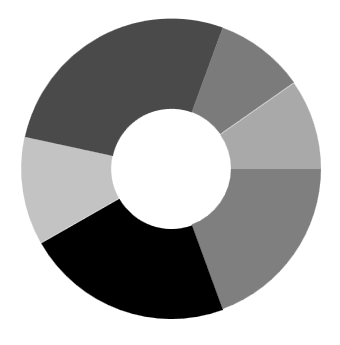The Doughnut also having coefficient property, `DoughnutCoefficient` which defines the inner circle. Also it has `DoughnutSize`, used to define the size for this series like `PieCoefficient` in PieSeries.

``````<chart:DoughnutSeries DoughnutCoefficient="0.7"

XBindingPath="Category" ItemsSource="{Binding Tax}"

YBindingPath="Percentage" />``````
``````DoughnutSeries series = new DoughnutSeries()
{

ItemsSource = new ViewModel().Tax,

XBindingPath = "Category",

YBindingPath = "Percentage",

DoughnutCoefficient = 0.9
};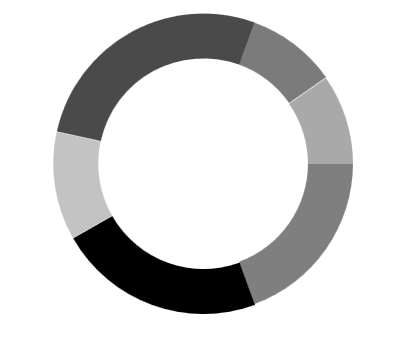Size

The size of doughnut series can be customized by using its `DoughnutSize` property. The following code illustrates how to use the property in series.

``````<syncfusion:SfChart>

<syncfusion:DoughnutSeries DoughnutSize="0.8"/>

<syncfusion:DoughnutSeries DoughnutSize="0.8"/ >

</syncfusion:SfChart>``````
``````DoughnutSeries doughnut = new DoughnutSeries();

doughnut.DoughnutSize = 0.8;

DoughnutSeries doughnut1 = new DoughnutSeries();

doughnut1.DoughnutSize = 0.8;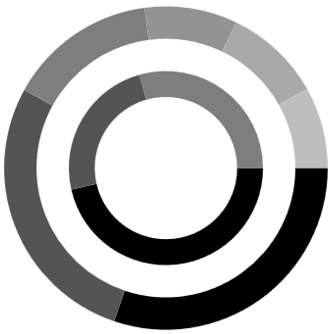Hole Size

`DoughnutHoleSize` is an attachable property. It gets or sets the double value, which is used to customize the doughnut hole size. Its value ranges from 0 to 1, and it can be set as shown in the following code example.

``````<Chart:SfChart Chart:DoughnutSeries.DoughnutHoleSize="0.2">

</chart:SfChart>``````
``````SfChart chart = new SfChart();

DoughnutSeries.SetDoughnutHoleSize(chart, 0.2);``````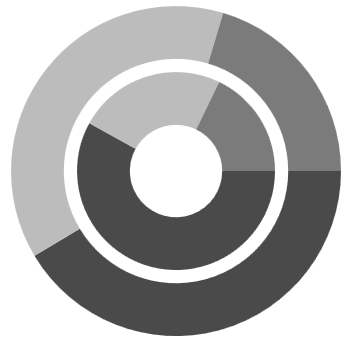## Semi Pie and Doughnut

By using custom `StartAngle` and `EndAngle` properties, you can draw pie series in different shapes such as semicircular or quarter circular series.

``````<syncfusion:PieSeries x:Name="PieSeries" StartAngle="180" EndAngle="360"

XBindingPath="Utilization"

YBindingPath="ResponseTime"

ItemsSource="{Binding}"/>``````
``````PieSeries series = new PieSeries()
{

ItemsSource = new ViewModel().Value,

XBindingPath = "Utilization",

YBindingPath = "ResponseTime",

StartAngle = 180,

EndAngle = 360

};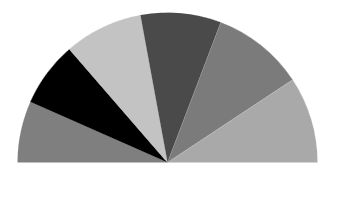``````<syncfusion:DoughnutSeries StartAngle="180" EndAngle="360"

XBindingPath="Utilization"

YBindingPath="ResponseTime"

ItemsSource="{Binding}"/>``````
``````DoughnutSeries series = new DoughnutSeries()
{

ItemsSource = new ViewModel().Value,

XBindingPath = "Utilization",

YBindingPath = "ResponseTime",

StartAngle = 180,

EndAngle = 360

};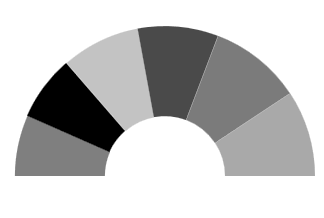## Stacked doughnut

Doughnut segments can be separated as individual circles using the `IsStackedDoughnut` property. The following properties are used to customize the stacked doughnut chart:

`CapStyle` - Specifies the shapes of the start and end points of a circular segment. The supported values are `BothFlat`, `BothCurve`, `StartCurve`, and `EndCurve`. The default value of the this property is BothFlat.
`SegmentSpacing` - Changes the spacing between two individual segments. The default value of spacing is 0, and the value ranges from 0 to 1. Here, 1 represents 100%, and 0 represents 0% of the available space.
`MaximumValue` - Represents the entire span of an individual circle. The default value of the this property is double.NaN.
`TrackColor` - Changes the color of the track area.
`TrackBorderColor` - Changes the color of the track border.
`TrackBorderWidth` - Changes the width of the track border.

``````<chart:SfChart>
…
<chart:DoughnutSeries XBindingPath="Category" YBindingPath="Expenditure" ItemsSource="{Binding ExpenditureData}"
IsStackedDoughnut="True" CapStyle="BothCurve" SegmentSpacing="0.2"
MaximumValue="100">
</chart:DoughnutSeries>

</chart:SfChart>``````
``````SfChart chart = new SfChart();

DoughnutSeries doughnutSeries = new DoughnutSeries()
{
XBindingPath = "Category",
YBindingPath = "Expenditure",
ItemsSource = new ViewModel().ExpenditureData,
IsStackedDoughnut = true,
CapStyle = DoughnutCapStyle.BothCurve,
SegmentSpacing = 0.2,
MaximumValue = 100
};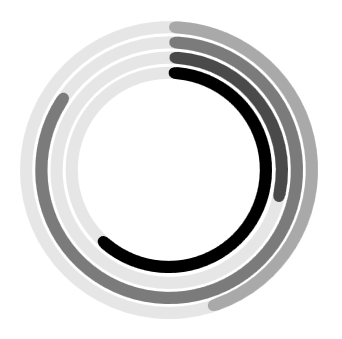### Add content to the center of doughnut chart

You can add any content to the center of the doughnut chart using the `CenterView` property of `DoughnutSeries`. The binding context of the `CenterView` will be the respective `DoughnutSeries`.

``````<chart:SfChart>
…
<chart:DoughnutSeries XBindingPath="Category" YBindingPath="Expenditure" ItemsSource="{Binding ExpenditureData}"
IsStackedDoughnut="True" CapStyle="BothCurve" SegmentSpacing="0.2"
MaximumValue="100">

<chart:DoughnutSeries.CenterView>
<ContentControl HorizontalAlignment="Center" VerticalAlignment="Center" >
<Image Source="/Image/Person.png" Width="164" Height="164"/>
</ContentControl>
</chart:DoughnutSeries.CenterView>

</chart:DoughnutSeries>

</chart:SfChart>``````
``````ContentControl centerView = new ContentControl()
{
Content = new Image()
{
HorizontalAlignment = HorizontalAlignment.Center,
VerticalAlignment = VerticalAlignment.Center,
Source = new BitmapImage(new Uri("Image/Person.png", UriKind.Relative)),
Width = 164,
Height = 164
}
};

doughnutSeries.CenterView = centerView;``````## Explode Segments

The following properties are used to explode the individual segments in Pie and Doughnut.

Explode Index

``````<syncfusion:PieSeries x:Name="PieSeries" ItemsSource="{Binding}"

ExplodeIndex="2"

XBindingPath="Utilization"

YBindingPath="ResponseTime" />``````
``````PieSeries series = new PieSeries()
{

ItemsSource = new ViewModel().Data,

XBindingPath = "Utilization",

YBindingPath = "ResponseTime",

ExplodeIndex = 2,

};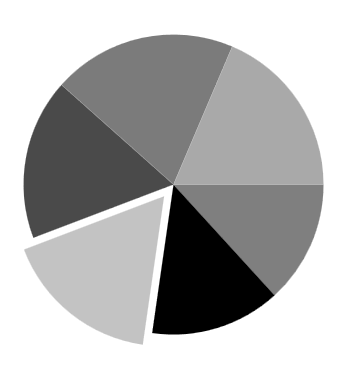NOTE

We have defined ExplodeRadius as 30, by default its value is zero. So you need to define explode, when you set ExplodeIndex or ExplodeAll.

Explode All

``````<chart:PieSeries  ExplodeAll="True"

XBindingPath="Category"

ItemsSource="{Binding Tax}"

YBindingPath="Percentage">``````
``````PieSeries series = new PieSeries()
{

ItemsSource = new ViewModel().Tax,

XBindingPath = "Category",

YBindingPath = "Percentage",

ExplodeAll = true,

};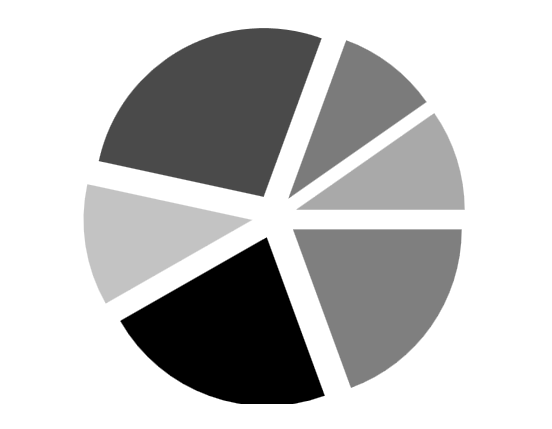`How to create Pie Chart in C# WPF`